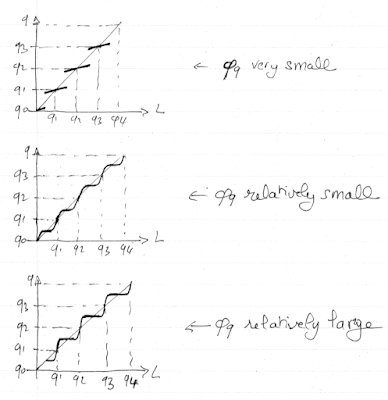## Tuesday, May 15, 2018

### Non Photorealistic Rendering - Pseudo-Quantization

This is called pseudo-quantization instead of quantization because it is based solely upon the luminance channel (of the CIE-Lab color space). The goal here is to simulate cel shading aka toon shading (just like it is done in cartoons). Of course, you'd better be not too picky otherwise you are going to be quite disappointed by the results. The main issue is how to handle the discontinuities between the quantized values: you don't want big color jumps especially in areas where the luminance gradient is small. If the quantized values are not smoothed in any way, then you have in your hands a hard quantization. If some efforts are made to smooth the transitions, it is soft quantization we are talking about.

A pioneer in this technique is Holger Winnemöller. So, let's take a look at "Real-Time Video Abstraction" and see how he handles quantization.Quantized values for the luminance using a very small phi_q, a relatively small phi_q, and a relatively large phi_q.

Note that if phi_q is set to 0, the quantized values are q0, q1, q2, etc. As phi_q increases though, the quantized values change to q0+delta_q/2, q1+delta_q/2, q2+delta_q/2, etc. Winnemöller suggests using between 8 to 10 bins and a phi_q between 3.0 and 14.0. The transitions between quantized values are much smoother when phi_q is smaller (good!) but the (horizontal) steps are much shorter (not so good!).

Let's see the results of Winnemöller soft quantization on a real image ...

Yeah, there's definitely quantization happening but not sure you are gonna be able to see the difference between the quantized images. Let's zoom in the upper right!

Clearly, the quantized image with phi_q = 3.0 has a smoother transition between flat areas of color than the quantized image with phi_q = 14.0. Kinda looks like the quantized image with phi_q = 3.0 is an anti-aliased version of the quantized image with phi_q = 14.0.

In the paper, Winnemöller is not satisfied with a uniform phi_q. Clearly, phi_q should be a function of the luminance gradient. Indeed, you kinda want phi_q to be relatively small when the luminance gradient is small and you want phi_q to be relatively large when the luminance gradient is large. All you have to do is compute the magnitude of the luminance gradient, clamp it on either end (min and max), and linearly interpolate phi_q between phi_q_min = 3.0 (corresponds to the min luminance gradient magnitude) and phi_q_max = 14.0 (corresponds to the max luminance gradient magnitude).